Next: 1. Introduction Up: Dissertation R.L. de Orio Previous: List of Tables

# List of Symbols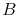Bulk modulus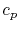Specific heat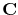Elasticity tensorAtomic concentration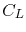Concentration of lattice sites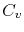Vacancy concentration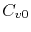Equilibrium vacancy concentration in the absence of stress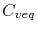Equilibrium vacancy concentration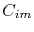Trapped vacancy concentration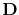Vacancy diffusivity tensor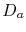Atomic diffusion coefficient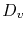Vacancy diffusion coefficient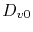Pre-exponential factor for vacancy diffusivity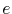Elementary chargeYoung modulus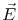Electric field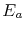Activation energy for diffusion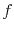Vacancy relaxation factor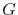Vacancy generation/annihilation term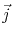Current density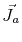Atomic flux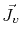Vacancy flux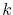Boltzmann constant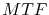Mean time to failure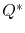Heat of transport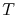Temperature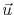Displacement vector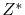Effective valence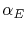Linear temperature coefficient of the electrical conductivity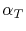Linear temperature coefficient of the thermal conductivity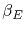Quadratic temperature coefficient of the electrical conductivity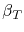Quadratic temperature coefficient of the thermal conductivity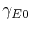Electrical conductivity at room temperatureThermal conductivity at room temperature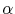Thermal expansion coefficient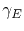Electrical conductivity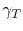Thermal conductivity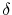Grain boundary/interface width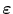Total strain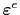Elastic strain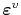Electromigration induced strain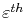Thermal strain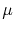Chemical potential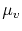Vacancy chemical potential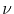Poisson ratio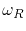Vacancy release rate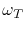Vacancy trapping rate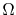Atomic volume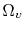Vacancy volume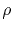Electric resistivity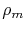Material density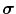Mechanical stress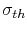Critical stress for void nucleation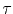Vacancy relaxation timeNext: 1. Introduction Up: Dissertation R.L. de Orio Previous: List of Tables

R. L. de Orio: Electromigration Modeling and Simulation